# Chain Rule of Calculus for Neural Networks and Deep Learning

#### Introduction

In Machine Learning, our primary goal is to find the most optimum values for the learnable parameters such that the value of the cost function becomes minimum. To find these optimum values, machines start with random values (as initialization) for these learnable parameters and then slowly update these parameters by sensing the direction (increase or decrease) in which the update should happen.

ML algorithms use optimization algorithms, like Gradient Descent, Stochastic Gradient Descent, or Adam, to find this direction. All these optimization algorithms use gradients to sense the direction of the update. In this article, we will use the Gradient Descent algorithm to find how gradients help backpropagation or update the parameters when training ML models.

#### Key takeaways from this blog

After going through this article, we will be able to understand the following things:

• Why do we calculate derivatives or partial derivates in Neural Networks?
• Why is derivative calculation considered difficult for ANNs compared to traditional ML algorithms?
• Use of chain rule while performing backpropagation in ANNs.
• Common mistakes while applying the chain rule.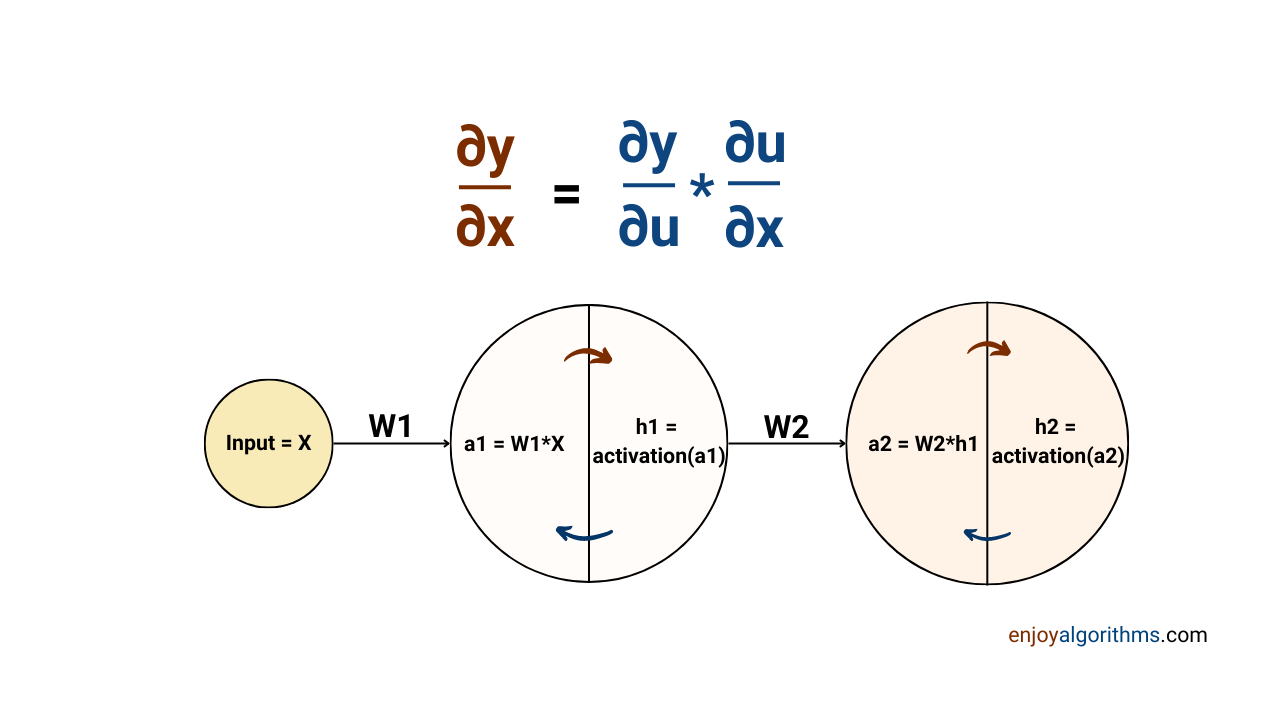The chain rule helps in finding the partial derivative of the cost function with respect to the learnable parameters. But before this discussion, we should know why we calculate the derivative or partial derivative of the cost function.

#### A Quick Recap on Derivative of any Mathematical Function

Derivates of a function at any point describe the slope of that function at that point. For example, the image below shows the derivate of f(x) = x², at x = 5. From the derivative theory, differentiating x² with respect to x will produce 2*x. So, at x=5, the derivative of f(x) = x² will be 2*5 = 10, a positive value. The sign of this derivative at a certain point conveys critical information about the curve at that point.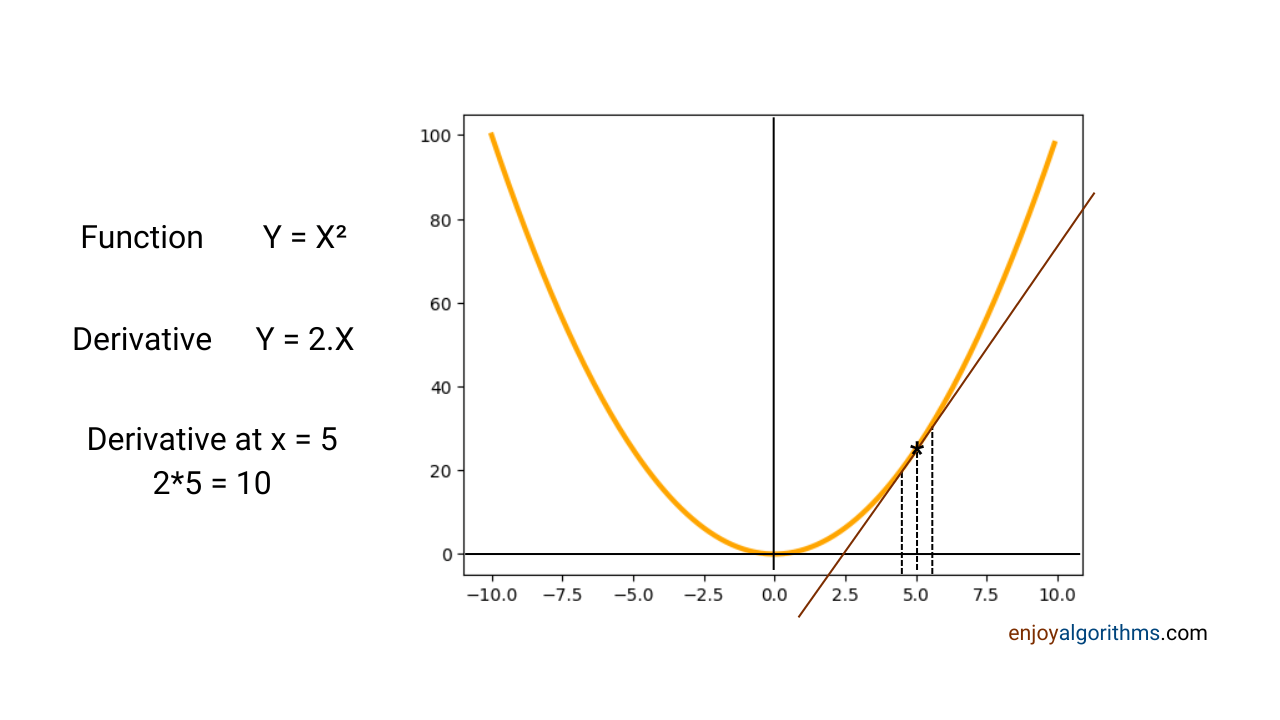• A positive slope represents a condition where an increase in the value of x will increase the value of f(x). If we decrease the value of x, the value of f(x) will also decrease.
• A negative slope represents a condition where an increase in the value of x will decrease the value of f(x). If we decrease the value of x, the value of f(x) will increase.

#### Why do we need derivatives in Machine Learning?

In Machine Learning, f(x) is the cost function, x is the parameter, and the goal is to achieve that value of x, for which f(x) is the minimum. Optimization algorithms start with a random value for x and then use the cost function's derivatives to understand whether it should increase or decrease the parameter's value to achieve the minimum.

In Gradient Descent, the parameter update happens in this way: θ:= θ — α*d(J(θ))/d(θ). Here, α is known as the learning parameter, and one can find the requirement/effects of α in our blog on the gradient descent algorithm. For example, in the image below, θ1' is the desired parametric value machines want to learn, and the updation equation is: θ1:= θ1 — α*d(J(θ1))/d(θ1).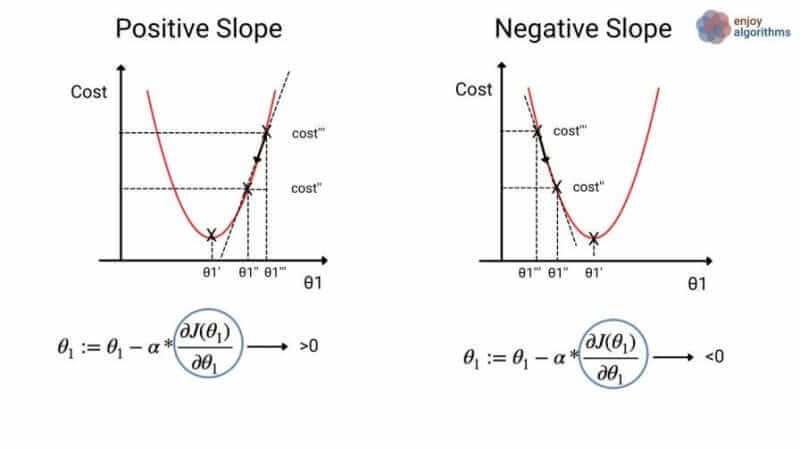In starting, the machine will randomly pick any 'θ1', and only three possible scenarios can be expected:

1. θ1 > θ1': The optimization algorithm will ask ML algorithms to reduce the value for θ1 so that θ1 → θ1'.
2. θ1 < θ1': The optimization algorithm will ask ML algorithms to increase the value for θ1 so that θ1 → θ1'.
3. θ1 = θ1': No update in the parameter value is required. ML process will be completed.

The update parameter uses a negative (-) of the derivative. Hence, in the case of a positive slope, the value for that parameter will decrease; if the slope is negative, the value for the parameter will increase.

In most machine learning use cases, multiple learnable parameters are involved in the learning process. Machines intended to find the optimum value for all those parameters. If any ML model involves "n" parameters, all these parameters will somehow affect the cost function. So, we can represent the cost function as J(θ1, θ2, …, θn). All these parameters need to be updated in the right direction to find the minimum value for J.

But if we calculate the derivative of this cost function (which depends on' n' variables), all constituent variables will start affecting the update process for one variable. ML algorithms will find it difficult to sense whether to increase or decrease the value. That's why the concept of partial derivative comes into the picture.

#### Why do we need derivatives in Machine Learning?

While calculating the partial derivative of a function with respect to any one parameter out of multiple parameters, we treat that parameter as the only variable affecting that function at that time. Rest all parameters are considered to be constant for that function. This helps to focus on one parameter during the updation process, and that's why we use partial derivatives in machine learning.

``````Normal derivative --> d(J(θ1, θ2, …, θn))/dθ1 = d((J(θ1, θ2, …, θn))/dθ1 * dθ2/dθ1 * ... * dθn/dθ1

Partial derivative --> d(J(θ1, θ2, …, θn))/dθ1 = d((J(θ1, θ2, …, θn))/dθ1``````

#### Why do we need the chain rule to find the derivative of the cost function in ANN?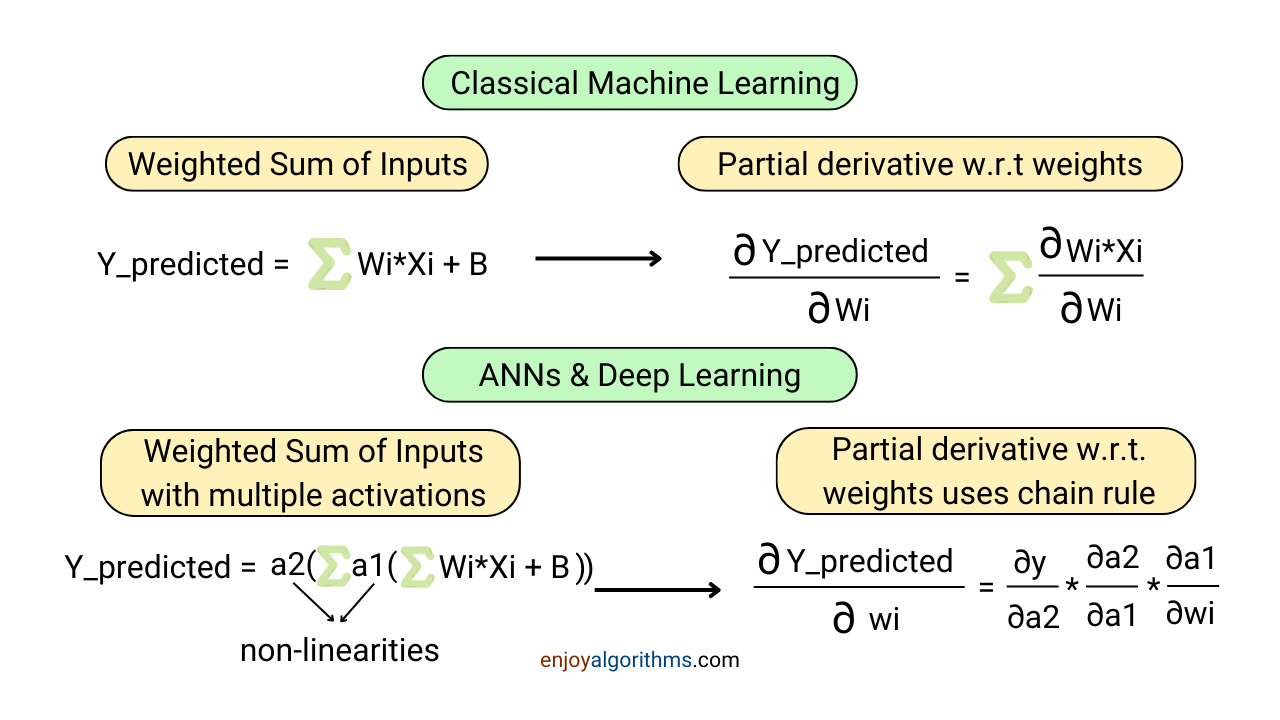Calculating partial derivates in the case of classical machine learning was trivial as the transformation of input parameters only involved the weighted sum of input features. However, in the case of ANNs or other deep learning algorithms (CNNs, RNNs, or Transformers), the input data passes through one or multiple hidden layers containing non-linear activation functions.

If we have even one hidden layer in the network, the output from the output layer will get nested (meaning f(g(x))) as it will receive input from the hidden layer. In mathematics, a nested function is known as the Composite function, and the chain rule is the only way to find the derivative of the composite function. Let's understand these two terms in detail.

#### What are Composite Functions?

A function is composite if it can be written as f(g(x)), something like a function of a function. Here, f(x) is the "Outer" function, and g(x) is the "Inner" function. For example, sin(x²) is a composite function, where g(x) = x² is the inner function and f(x) = sin(x) is the outer function. If we composite these two as f(g(x)), it will result in a composite function sin(x²).

Finding the derivative of this composite function depends significantly on correctly recognizing the composite function and its inner and outer functions. If we fail to identify a function that is not composite, it will produce wrong results when differentiated. Transcendental functions (Trigonometric or logarithmic functions) are among the most confusing functions where people get confused. For example, log(sin(x)) is a composite function with inner function sin(x) and outer function log(x).

The chain rule is a method to find the derivative of the composite function. So, let's understand it in more detail.

### Chain Rule

The chain rule says: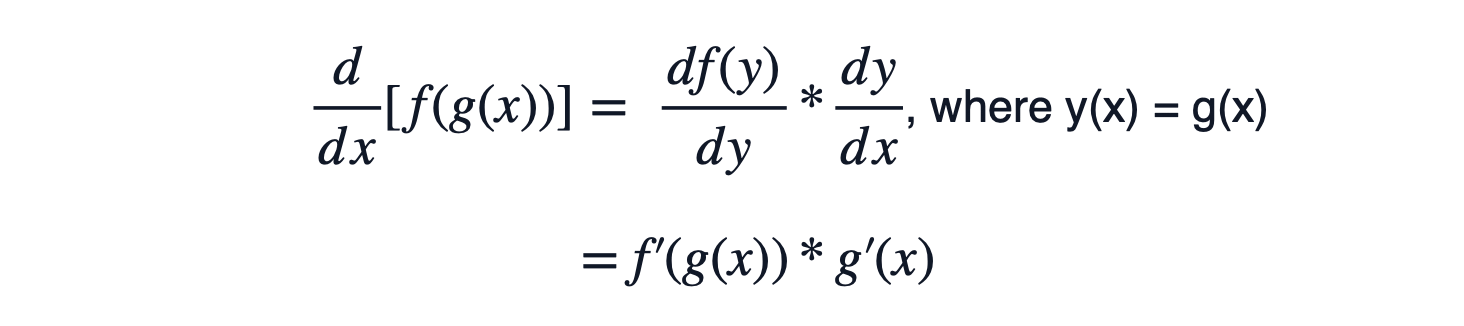In the equation above, f' (x) is the derivative of function' f' with respect to 'x'. One can see that the roles of inner and outer functions are different in the above equation. Hence, getting an accurate idea of inner and outer functions from a composite function will be much needed.

#### A common example of the chain rule

Let's see an example to see the working of the chain rule on a composite function. In the image below, calculations suggest that the derivative of composite function sin(cos(x)) will result in -cos(cos(x))*sin(x) as the derivative of sin(x) gives cos(x) and the derivative of cos(x) gives negative of sin(x).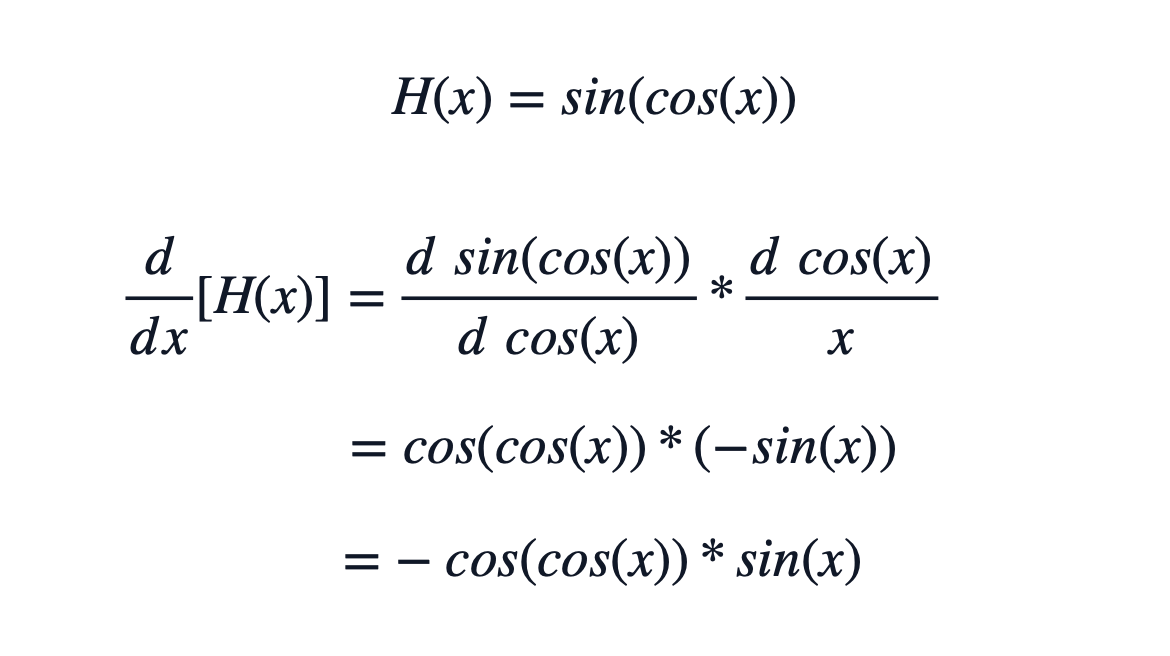#### Use of Chain Rule in Artificial Neural Networks

As discussed earlier, the partial derivative for the cost function with respect to the parameters will be required. Let's take an example of a neural network containing one hidden layer with a single node and sigmoid as the activation function. The output layer is also using the sigmoid as the activation function. If we choose the cost function as the difference between actual and predicted values, it will look like this: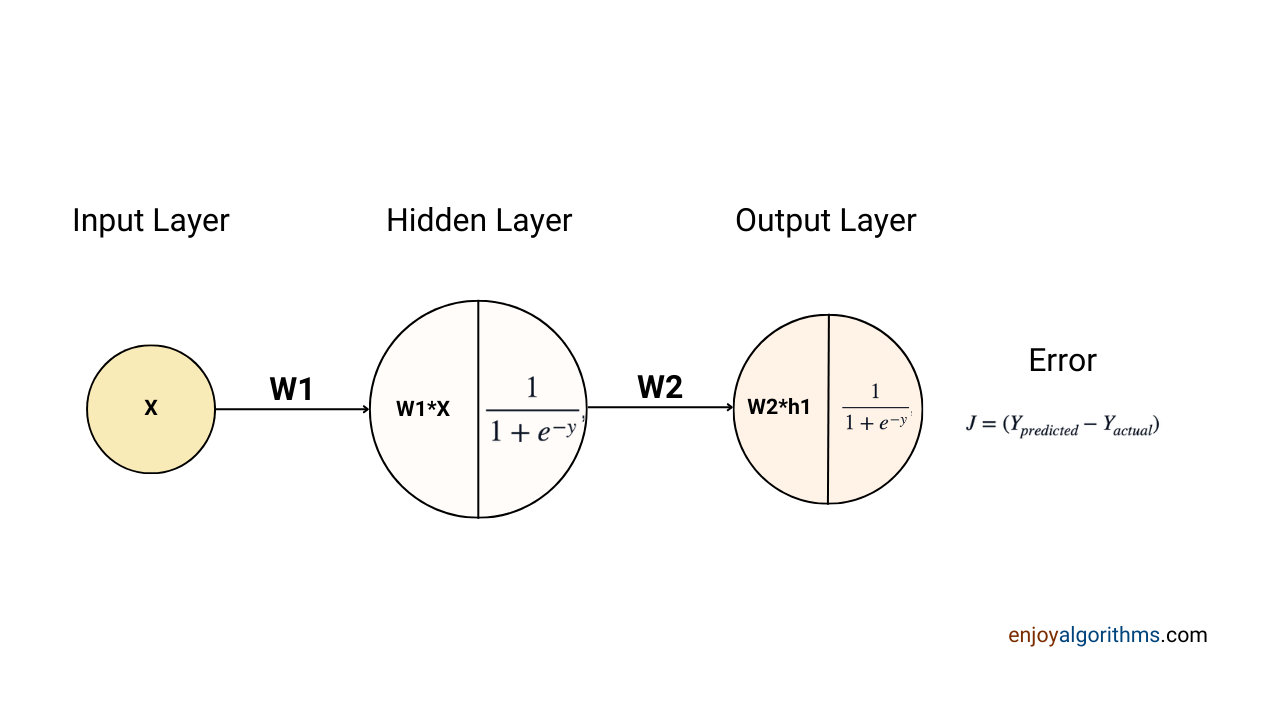Now, let's formulate the Y_predicted term in terms of input X and see the partial derivative of cost function J.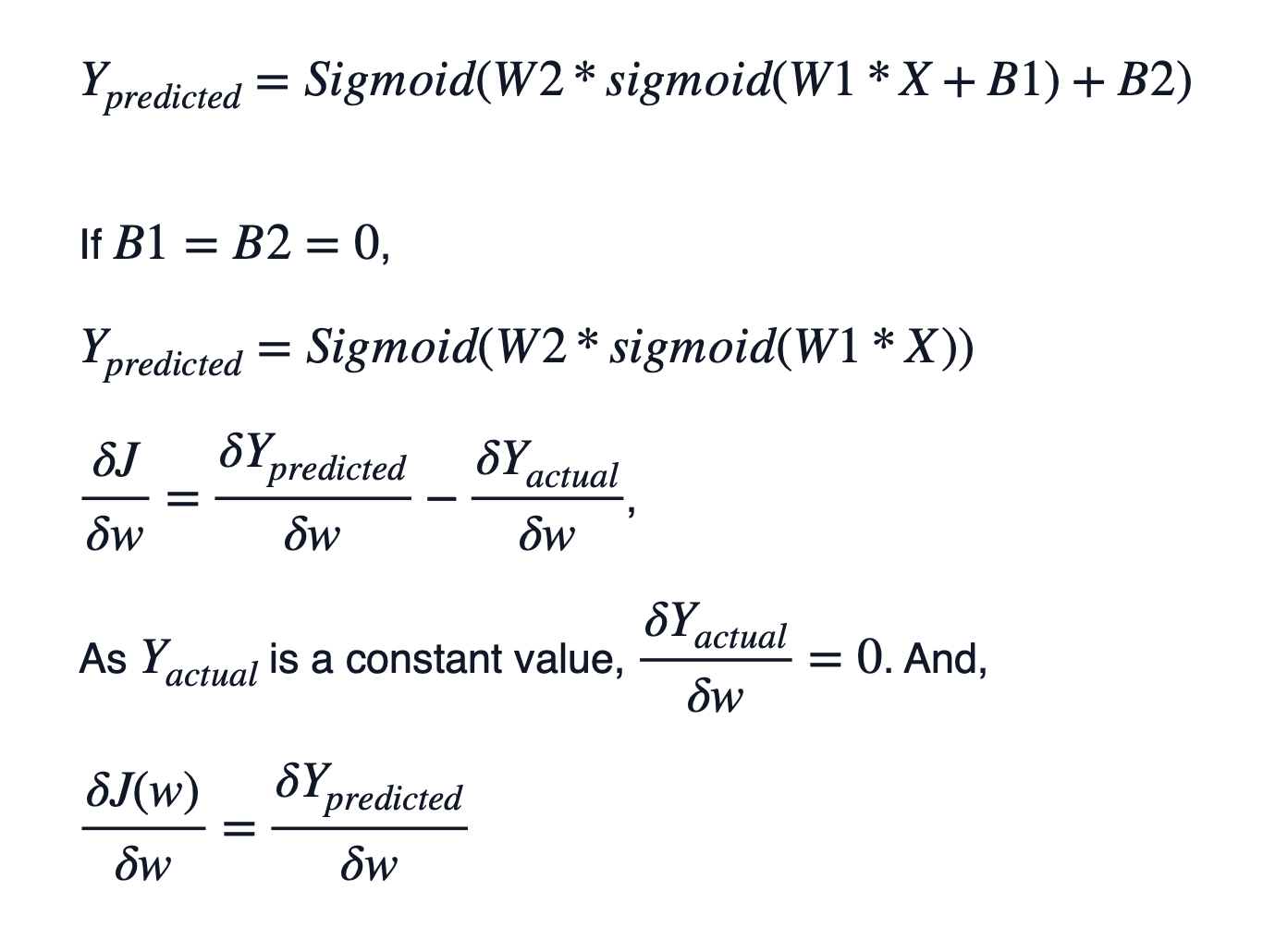If one notices carefully, the Sigmoid function is itself a composite function. Can you guess the inner and outer functions forming the sigmoid function?

The outer function in sigmoid is 1/x, and the inner function is 1 + e^-x. Even e^-x is another composite function with an inner function as -x and an outer function as e^x. If we differentiate e^-x, it will produce, (e’^-x * (-1)) → -e^-x.

The derivative of the sigmoid activation function will need a chain rule, and the same is shown in the image below.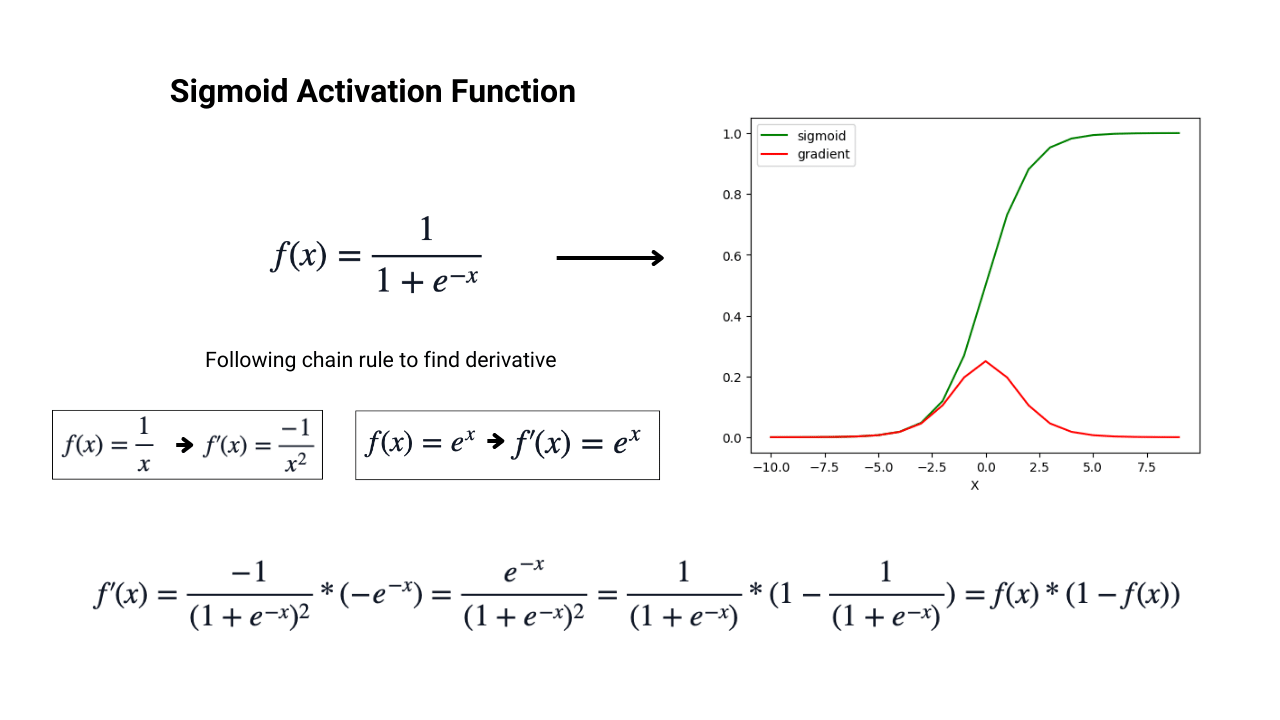The sigmoid derivative can be represented in the form of the original sigmoid function, which saves extra computation time and is considered the advantage of the sigmoid, which is discussed in our blog on activation functions for the hidden layers in neural networks.

Please note that the variable is not the input in calculating the derivative. We need to calculate the derivative of the cost function with respect to the weight values, which are W1 and W2 in the current scenario.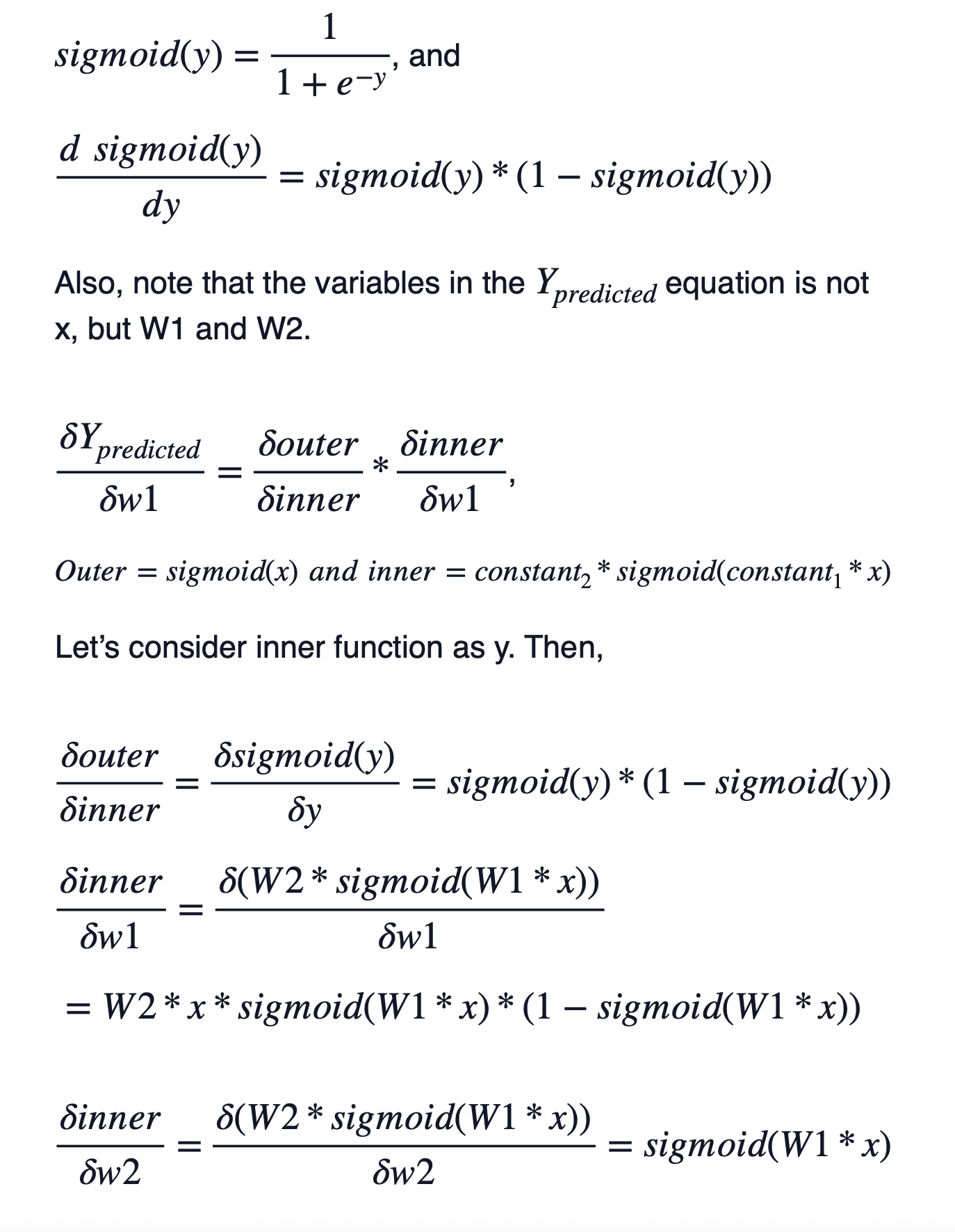Based on these derivative calculations, machines will update the weight values W1 and W2 and drive the cost function toward its minimum.

The knowledge of chain rule is essential to know the functioning of ANNs thoroughly. But, before closing this theory, let's discuss common mistakes one can make while calculating the chain rule.

#### Common Mistakes in applying the chain rule

• Failing to recognize whether a function is composite: It can sometimes be confusing to decide whether the function is composite, especially in the case of transcendental functions.
• Failing to recognize the correct inner and outer functions: The roles of inner and outer functions differ in the chain rule. For example, sin²(x) is a composite function with the inner function as sin(x) and the outer function as x². Derivative of sin²(x) will be 2*sin(x)*cos(x). But if someone uses x² as inner and sin(x) as outer, derivative results would be cos(x²)*2x, which is very different.
• Forget to multiply the derivative of the inner function: In the equation of the chain rule, we saw that d(f(g(x)))/dx = f' (g(x))*g' (x). Sometimes, especially when differentiating f(g(x)) is lengthy, we forget to multiply the second derivative term of g' (x), which will give wrong results.
• Calculating f' (g' (x)): It is one of the most common mistakes while performing the chain rule as d(f(g(x)))/dx gives f' (g(x))*g' (x), not f' (g' (x)).

### Conclusion

In this article, we discussed the chain rule of calculus and its use case in Machine Learning and Artificial Neural Networks. The chain rule is used to find the derivative of the composite function in ANNs and helps update the parameters in the right direction. While discussing this, we also learned about common mistakes one can make when applying chain rules on complex nested or composite functions. We hope you enjoyed the article and learned something new.

We hope you enjoyed the blog. If you have any queries or feedback, please write us at contact@enjoyalgorithms.com. Enjoy learning, Enjoy algorithms!

☆ 16-Week Live DSA Course
☆ 10-Week Live DSA Course